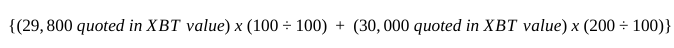Below calculation examples are using XBT values for XBT margined contracts. Similiar calculation applies for contracts margined in other relevant cryptocurrencies.

Note: On short XBT positions, it is necessary to round up the average satoshi value where long positions should be rounded down.

For example, the XBTUSD perpetual swap contract is quoted in USD. 29,800 XBT/USD means 29,800 USD per XBT. If we want to quote the price in XBT, it is calculated as below assuming the lot size is 100.

1. Divided by the XBTUSD price  100/29,800 = 0.00335570469

2. Round the value to 8 decimal places. 0.00335570 is the XBT value of 29,800 USD for 1 lot.As we now understand how to quote the price of the contract in XBT value. We will calculate the entry price in different cases.

Scenario 1: The order executed at the same price

You bought 100 contracts of XBTUSD Perpetual Swap contract, and your order filled for 100 contracts at \$29,800. In this case, you don’t need to calculate the average entry price. As you can see, the average entry price is \$29,800.

Conversely, you sold 100 contracts of the same contract and filled as above, and the average entry price is \$29,800 as well.

Scenario 2: The order executed at different prices

You bought 300 contracts of XBTUSD and they are filled for 100 contracts at \$29,800, and 200 contracts at \$30,000. (XBT lot size = 100 contracts)You need to calculate the average entry price in XBT, then convert to the USD price.

1. You quote \$29,800 in XBT value.
100/29,800 = 0.00335570469
We round the value down to 8 decimal places.
The XBT value of \$29,800 is 0.00335570 XBT.

2. You quote \$30,000 in XBT value.
100/30,000 = 0.00333333333
We round the value down to 8 decimal places.
The XBT value of \$30,000 is 0.00333333 XBT.

3. To get the average price, you can just add the satoshi value and divide by the number of lots of contracts.
{(\$29,800 quoted in XBT value)*(100/100) + (\$30,000 quoted in XBT value)*(200/100)}
= {0.00335570 * (100/100) + 0.00333333 * (200/100)} = 0.01002236

4. Average price in satoshi per lot after the first 2 buy orders, round down:
(0.0033557 + 0.00666666) / (300/100) = 0.00334078

5. Average price in USD = 1/ (0.00334078/100) = 29933.13